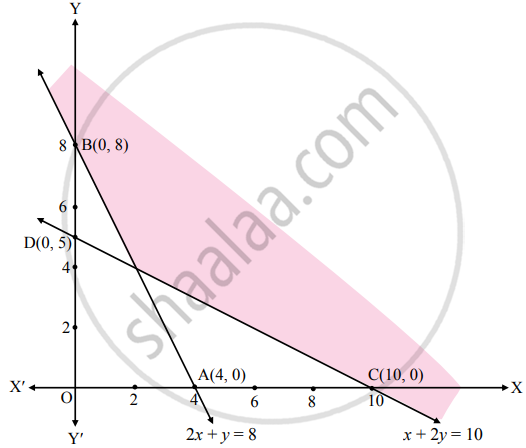# Solve the following system of inequalities graphically 2x + y ≥ 8, x + 2y ≥ 10 - Mathematics and Statistics

Graph

Solve the following system of inequalities graphically.
2x + y ≥ 8, x + 2y ≥ 10

#### Solution

To find graphical solution, construct the table as follows:

 Inequation Equation Double Intercept form Points (x, y) Region 2x + y ≥ 8 2x + y = 8 "x"/4 + "y"/8 = 1 A (4, 0),B (0, 8) 2(0) + 0 ≱  8∴ 0 ≱  8∴ non-origin side x + 2y ≥ 10 x + 2y = 10 "x"/10 + "y"/5 = 1 C (10, 0),D (0, 5) 0 + 2(0) ≱ 10∴ 0 ≱  10∴ non-origin sideThe shaded portion represents the graphical solution.

Concept: Graphical Solution of Linear Inequality of Two Variable
Is there an error in this question or solution?
Chapter 8: Linear Inequations - Miscellaneous Exercise 8 [Page 122]

#### APPEARS IN

Balbharati Mathematics and Statistics 2 (Commerce) 11th Standard HSC Maharashtra State Board
Chapter 8 Linear Inequations
Miscellaneous Exercise 8 | Q 7 | Page 122
Share